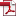• KSII Transactions on Internet and Information Systems
Monthly Online Journal (eISSN: 1976-7277)

Mathematical Analysis Power Spectrum of M-ary MSK and Detection with Optimum Maximum Likelihood

Vol. 15, No. 8, August 31, 202110.3837/tiis.2021.08.011, Download Paper (Free):Abstract

In this paper, the power spectral density(PSD) for Multilevel Minimum Shift Keyed signal with modulation index h=1/2 (M-ary MSK) are derived using the mathematical method of the Markov Chain model. At first, according to an essential requirement of the phase continuity characteristics of MSK signals, a complete model of the whole process of signal generation is built. Then, the derivations for autocorrelation functions are carried out precisely. After that, we verified the correctness and accuracy of the theoretical derivation by comparing the derived results with numerical simulations using MATLAB. We also divided the spectrum into four components according to the derivation. By analyzing these figures in the graphic, each component determines the characteristics of the spectrum. It is vital for enhanced spectral characteristics. To more visually represent the energy concentration of the main flap and the roll-down speed of the side flap, the specific out-of-band power of M-ary MSK is given. OMLCD(Optimum Maximum Likelihood Coherent Detection) of M-ary MSK is adopted to compare the signal received with prepared in advance in a code element T to go for the best. AndM-ary MSKBER(Bit Error Rate) is compared with the same ary PSK (Phase Shift Keying) with M=2,4,6,8. The results show the detection method could improve performance by increasing the length of L(memory inherent) in the phase continuity.